# Physics Topic 6A- Properties of waves

• Created by: millieray
• Created on: 05-03-18 14:57

## What Is A Wave?

• A wave is an oscillation (vibration) that transfers energy without transfering any matter, by mkaing the particles of the substance (or field) that it is travelling through oscillate.
• Waves can be iether transverse or longitudinal.
1 of 32

## Transverse Waves

• Waves transfer energy in the same direction that they travel.
• In transverse waves the oscillations are perpendicular (at 90°) to the direction of enregy transfer of the wave.
• Examples:
• light and all other electromagnetic waves
• ripples on wtaer
• waves on strings
• waves on a spring when wiggled up and down
2 of 32

3 of 32

## Longitudianl Waves

• Longitudinal waves have oscillations along the smae line as they travel.
• They have areas of compression, in which the particles are bunched together, and areas of rarefaction, in which the particles are spread out.
• In longitudinal waves oscillations are parallel to the direction of energy transfer of the wave.
• Examples:
• Sound waves
• A spring when you push the end
4 of 32

5 of 32

## Waves And Matter

• All waves transfer energy in the direction in which they are travelling, but they don't transfer matter.
• When waves travel through a medium (a material), such as air or water, the particles of the medium oscillate and transfer energy between each pother, but overall the particles stay in the same place- only energy is transferred.
• Some examples:
• Ripples on a water surface cause floating objects, e.g. twigs or birds, to just bob up and down. They don't move the object across the water to the edge. This is evidence that the wave travels but not the water.
• If you strum a guitar string and create sound waves, the sound waves don't carry the air away form the guitar to create a vacuum (completely empty space).
6 of 32

## Representing Waves

• A wave can be represented on a set of axes - a line is drawn to show the displacement of the particles from their undisturbed positions at a moment in time.
• It is as if a transverse wave had been set up in a piece of string and a 'snapshot' taken of it.
• Crests adn troughs are just points of maximum positive and maximum negative displacement from the particle's rest position.
7 of 32

## Representing Waves Diagram8 of 32

## Wave Measurements

• The amplitude of a wave is the maximum displacement of a point on the wave from its undisturbed (or rest) position. In other words it's the distance from the undisturbed position to a crest or a trough.
• The wavelength is the distance between the same point on two adjacent waves. So on a transveres wave it may be the distance between the crest of one wave and the crest of the next wave.
• Frequency is the number of complete waves passing a certain point per second. Frequency is measured in hertz (Hz). 1 Hz is 1 wave per second.
9 of 32

## The Period Of A Wave

• The period of a wave is the amount of time it takes for a full cycle of the wave to be completed.
• In other words, it's the length of time between one crest passing a point and the next crest passing the same point.
• You can find the period of a wave from the frequency using the equation:
• period (s) = 1/frequency (Hz)              OR              T=1/f
10 of 32

## Wave Speed

• The wave speed is the speed at which energy is being transferred (or the speed the wave is moving at).
• You use this equation, called the wave equation, to work it out:
•  Speed (m/s) = frequency(Hz) x wavelength(m)
• OR
• v=fλ
11 of 32

## Measuring The Speed Of Sound In Air

• To measure the speed of sound, you need to find the frequency and the wavelength of a sound wave- you can then use the wave equation.
• You can generate a sound wave with a specific frequency by attaching a signal generator to a speaker. This sound wave can then be detected by microphones, which convert it to a trace on an oscilloscope.

Method:

• Set up your equipment as shown on the next card, with both microphones next to the speaker. The detected wave at each microphonecan be seen as a seperate wave on the oscilloscope.
• Slowly move one microphone away from the speaker. Its wave will shift sideways on the oscilloscope. Keep moving it until the two waves on the oscilloscope are alligned once more. At this point the microphones will be exactly one wavelength apart, so measure the distance between them.
• You can then use the formualr to find the speed- the frequency will be equal to the frequency set by the signal generator.
• The speed of sound in air is around 330m/s.
12 of 32

## Measuring The Speed Of Sound In Air- Equipment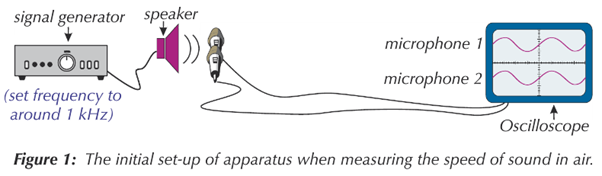[Image_Link]data:image/png;base64,iVBORw0KGgoAAAANSUhEUgAAAloAAACgCAIAAABbr1XrAAAgAElEQVR4nOydZXQcyZbnPbMzZz/s2T17dnZnXs+b97rtZnyNdtuWGSXZlixmxhIzMzMzMzOzLIYSM5dUKknFKuaK/ZByWZZk2W5bhnb+Tpw+zsjMiMhUdf3r3rg34hSAgYGBgYH54Dn1tgcAAwMDAwPz9oHlEAYGBubEYbN5DAaHy+UfqOdweAdqWCwuk8l5A0Pa2CAZGeaNj6MBAEKhUCAQvoFO3zAv9VCwHMLAwMCcOAP9q7a2pSPI9f2VVVUT+flD+2uWlrA+3nXT05g3MKShobXfzwZOTm4CABobpry9axmMNyHDb4zW1jlPjxoikf6C18NyCAMDA3PiLC7u3L0T3fVoUVSDw1Hv3I7y863bb8EkJna5u1Xz+YI3MKSiQqSBfg6JxAAAlJWNWloU02jsN9DvG6OmZtLMtBCHo73g9bAcwsDAwJw4BAL97p3ohvppUU1R0bCsbJKdbRmLxYVqMJhdC/Oiubntw7cLX8Dnd6SIstkHnbEi3FyrA/wbhI+bPuy2fRZv16364uN82YthOYSBgYE5cahU1oP7ccUlI9AhGk0OCW7OyOhTUU6jUJhQ
13 of 32

## Measuring The Speed Of Water Ripples- Required Pra

• Use a signal generator to produce waves of a known frequency and attach it to the dipper of a ripple tank.
• A ripple tank is a shallow glass tank used to show the properties of waves. The glass bottom of a ripple tank means a light can be shone on the tank from above to project the wave pattern onto a screen below. This makes it much easier to measure the waves without disturbing them.

Method:

• Set up the equipment as shown on the next card. Fil the ripple tank with water to a depth of around 5mm. Connect your dipper to the signal generator and set it off at a known frequency. Dim the lights and turn on the lamp- this shows a wave pattern made by the shadows of the wave crests on the screen below the tank.
• the distance between each shadow line is equal to one wavelength. As the wavelength is small, and the waves are constantly moving, it's tricky to measure just one of these accurately. Instead, measure the distance between shadow lines that are 10 wavelengths apart using a metre ruler. Then divide this distance by 10 to find the average wavelength. This is a suitable method for accurately measuring the wavelength of moving waves, or measuring small wavelengths.
• Then use the formula to find the wave speed.
14 of 32

## Measuring The Speed Of Water Ripples- Required Pra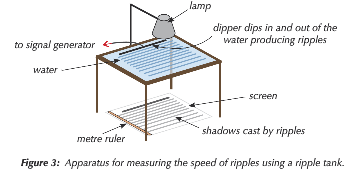15 of 32

## Observing A Wave On String - Required Practical 8

• You need a taut string and a piece of kit called a vibratiob transducer. A vibration transducer will convert an electrical signal from a signal generator into vibrations. When it's attached to a taut string, waves are formed on the string.
• To ensure the string is taut by connecting one end to the vibration transducer and passing it over a pulley with masses hanging from the other end. This will keep it pulled nice and tight.
• Set up the equipment as shown in the next card, then turn on the signal generator and vibration transducer. The string will start to vibrate.
• Adjust the frequency of the signal generator until there's a clear wave on the string. The frequency you need will depend on the length of string between the pulley and the transducer, and the masses you've used.
• You need to measure the wavelength of these waves. The best way to do this accurately is to measure the length of four or five half-wavelengths in one go, then divide to get the average half-wavelength. Then double this to get the average wavelength.
• The frequency of the wave is whatever the signal generator is set to. You can then find the speed of the wave usinng the formula.
16 of 32

## Observing A Wave On String - Required Practical 8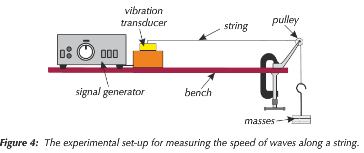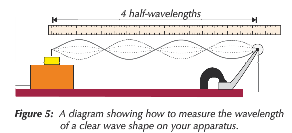17 of 32

## Waves At A boundary

• Waves travel through different materials. When they arrive at a boudnary between two differnet materials, 3 things can happen:
• Absorption- the waves may be absorbed by the material the wave is trying toi cross into. This transfers energy to the material's energy stores.
• Reflection- the waves may bounce back
• Transmission- the waves may be transmitted. This means that they carry on travelling through the material. However, they often undergo refraction.
18 of 32

## Refraction Basics

• When a waves reaches the boundary between two different materials, it can be transmitted through the new material. If it hits the boundary at an angle, it changes direction- it's refracted.
19 of 32

## Ray Diagrams

• A ray is a straight line showung the path a wave travels along.
• You can show the path taken by a light ray on a ray diagram.
• Draw the boundary between the two materials and then as in the normal (a straight line that is 90° to the boundary) normally hsown as a dotted line.
• Draw an incoming ray that meets the normal at the boundary.
• The angle between the ray and the normal is the angle of incidence.
• Now draw the refracted ray on the other side of the boundary. The anlge that the refracted ray makes with the normal is called the angle of refraction.
• The angle of refraction could be smaller that the angle of incidence or bigger.
If a ray travels along the normal and hits the boundary at 90°, it'll pass through without changing direction. The angles of incidence and refraction will both be 0°.
20 of 32

## Example Of A Ray Diagram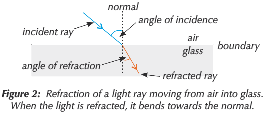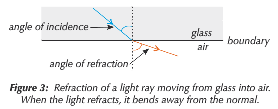21 of 32

## More On Refraction

• Refractio occurs because waves travel faster in some materials than others, so the speed of a wave can change as it crosses a boundary.
• When a wsve refracts, it changes speed, but its frequency remains the same.
• Speed, frequency and wavelength are all related by the wave equation so as speed changes and frequency stays the same, wavelength must change.
• If the speed of a wave increases so does the wavelength.
• If the speed of a wave decreases so does the wavelength.
• The optical density of a material is a measure of how quickly light travels through it - the higher the optical density, the slower light travels.
22 of 32

## Wavefronts

• Wavefronts are imaginary lines drawb trhough certain points on waves, e.g. through each crest.
• They're perpendicular to the direction in which the wave is moving.
• In the wavefront diagrm on the next card showing light waves travelling along a normal to a boundary. The wavefronts are closer together in the more optically dense material, sowing the decrease in wavelength.
• Wavefront diagrams are useful in explaining why refraction happens.
• When a wave crosses a boundary into a new substance at an angle to the normal, one end of it enters the new material before the rest of the wave. This means that the end changes speed before the rest of the wave, casuing the wave to change direction.
• Which way the wave beeds depends on two things:
• If a wave slows down at a boundary, it will bend towards the normal. Here the angle of refraction is smaller than the angle of incidence.
• If a wave speeds up at a boundary, it bends away from the normal. Here the angle of refraction is greater than the angle of incidence.
23 of 32

## Wavefront Pictures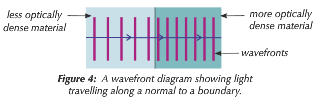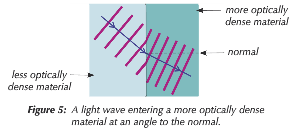24 of 32

## Wavefront Pictures Continued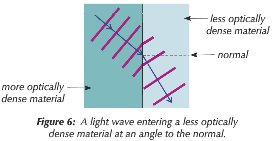25 of 32

## Ray Diagrams Of Reflection

• A wave can also be reflected when it hits a boundary of a different material.
• To draw a ray diagram showing reflection, you need to draw the incoming ray and the reflected ray. Also draw a normal.
• The angle of incidence is between the incoming wave and the normal.
• The angle of reflection is the angle between the reflected wave and the normal.
• The law of reflection applied to reflected wave is:
• angle of incidence=angle of reflection.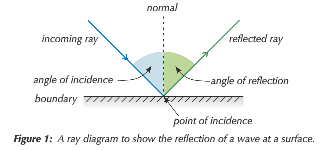26 of 32

## Types Of Reflection

• Waves are reflected differently at different boundaries:
• Specular reflection happens when parallel waves are reflected in a single direction by a smooth surface. e.g. when light is reflected by a mirror you get a nice clear reflection.
• Diffuse reflection is when parallel waves are reflected by a rough surface (e.g. a piece of paper) and the reflected rays are scattered in lots of different directions.
• The angle of incidence is still equal to the angle of reflection for each ray. However, the tiny bumps on the surface mean that the normal for each ray is different so the angle of incidence for each ray is different.
• When light is reflected by a rough surface, the surface appears matt and you don't get a clear reflection.
27 of 32

## Types Of Reflection- Pictures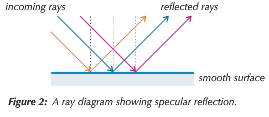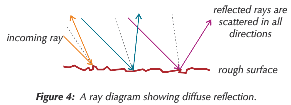28 of 32

## Experimenting With Light

• It's best to do these experiments in a dim room so you can clearly see the light rays.
• A ray box or laser should be used to produce thins light rays so you can easily see the middle of the ray when tracing and measuring angles from it.
29 of 32

## Investigating Refraction- Required Practical 9

• To investigate how boundaries refract different amounts of light you can look at how much a light ray is refracted when it passes from air into different transparent materials, such as glass.

Method:

• Place a transparent rectangular block on a piece of paper and trace around it. Then use a ray box to shine a ray of light at the middle of one side of the block.
• Trace the incident ray on the paper, and do the same for light ray that emerges on the other side of the block.
• Remove the block and, with a straight line, join up the lines for the incident ray and the emerging ray showing the path of the refracted ray through the block.
• Draw the normal at the point where the light ray entered and then measure the angle between the incident line and the normal (angle of incidence, I) and the angle between the refracted ray and the normal ( the angle of refraction,R).
• Repeat this experiment with a different material keeping the same incident angle throughout.
30 of 32

## Investigating Refraction- Required Practical 9-Equ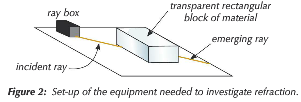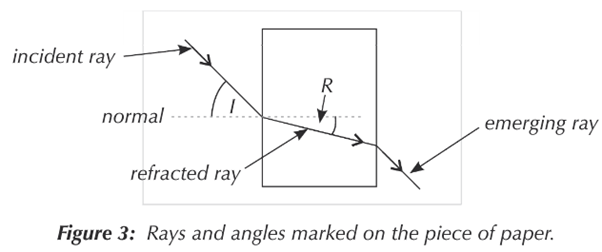31 of 32

## Investigating Reflection- Required Practical 9

• Different surfaces relfect light in different ways depending on how smooth they are.
• To investigate this, take a piece of paper and draw a straight line across it. Place a straight edged object on the paper so that it lines up with this line.
• Shine a ray of light at the object's surface and trace the incoming reflected light beams.
• Add in a normal line that meets the surface a the point of incidence.
• Draw and label the incident and reflected rays.
• Measure the angle of incidence and reflection.
• Measure and record the width of the reflected ray and make note of how bright it is.
• Repeat this for a range of objects.
•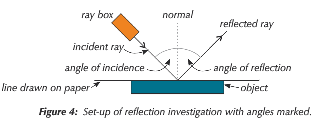32 of 32